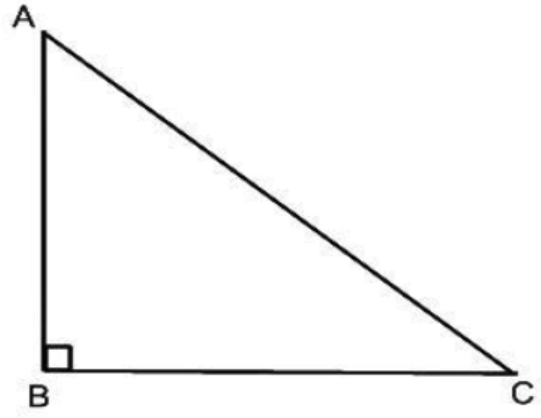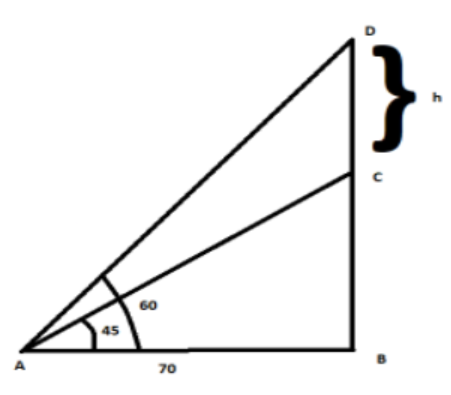QuestionAnswers

# A vertical tower standing on a horizontal plane is surmounted by a vertical flagstaff. At a point on the plane $70$ meters away from the tower, an observer notices that the angle of elevation of the top and the bottom of the flagstaff are respectively ${{60}^{\circ }}$ and ${{45}^{\circ }}$. Find the height of the flagstaff and that of the tower.Verified
149.7k+ views
Hint: Draw the figure of the situation that is given in the question and mark all the
distances and the angles given in the question. To solve this question, we must know that
$\tan$ of an angle is equal to the ratio of the perpendicular and the base of the triangle.

Before proceeding with the question, we must know that for an angle in a right angle triangle, the $\tan$ of that particular angle is equal to the ratio of the perpendicular and the base with respect to that angle of the triangle. If we consider a right angle triangle
$ABC$,If we consider $\angle C$, $\tan C=\dfrac{AB}{BC}................\left( 1 \right)$.
In this question, we have a vertical tower $BD$ which is standing on a horizontal ground. On this tower, a vertical flagstaff $CD$ is surmounted. An observer is standing on $A$ at a distance $70$ meters from the base of the tower i.e. $B$. For the observer at $A$, the angle of elevation of the bottom of the flagstaff i.e. $C$ is equal to ${{45}^{\circ }}$ and the angle of elevation of the top of the flagstaff i.e. $D$ is equal to ${{60}^{\circ }}$.The figure of the above situation is drawn below,Let us consider the height of the flagstaff $CD=h$.
In the question, we are required to find the height of the tower i.e. length of $BD$.
Applying formula $\left( 1 \right)$ on $\angle DAB={{60}^{\circ }}$, we get,
$\tan 60=\dfrac{BD}{AB}$
In the question, it is given that $AB=70$. Also, we have $\tan 60=\sqrt{3}$. Substituting this
in the above equation, we get,
\begin{align} & \sqrt{3}=\dfrac{BD}{70} \\ & \Rightarrow BD=70\sqrt{3} \\ \end{align}
Hence, the height of the tower $BD=70\sqrt{3}..........\left( 2 \right)$.
Also, in the question, we are required to find the height of flagstaff i.e.$CD$. For that, we will
first find $BC$.
Applying formula $\left( 1 \right)$ on $\angle CAB={{45}^{\circ }}$, we get,
$\tan {{45}^{\circ }}=\dfrac{BC}{AB}$
In the question, it is given that $AB=70$. Also, we have $\tan {{45}^{\circ }}=1$. Substituting
this in the above equation, we get,
\begin{align} & 1=\dfrac{BC}{70} \\ & \Rightarrow BC=70............\left( 3 \right) \\ \end{align}
Since $BD$ is a straight line, we can say,
\begin{align} & BC+CD=BD \\ & \Rightarrow CD=BD-BC \\ \end{align}
Since we have assumed the height of the flagstaff $CD=h$, we can substitute $CD=h$ in the
above equation. $BD=70\sqrt{3}$. Also, substituting $BD=70\sqrt{3}$ from equation $\left( 2 \right)$ and $BC=70$ from equation $\left( 3 \right)$ in the above equation, we get,
\begin{align} & h=70\sqrt{3}-70 \\ & \Rightarrow h=70\left( \sqrt{3}-1 \right) \\ \end{align}
Hence, the height of the vertical tower is $70\sqrt{3}$ and the height of the flagstaff is
$70\left( \sqrt{3}-1 \right)$. Since $\sqrt{3}=1.73$, the height of the vertical tower is
$70\times 1.73=121.10$ meters and the height of the flagstaff is $70\left( \sqrt{3}-1 \right)=70\left( 1.73-1 \right)=70\left( 0.73 \right)=51.10$ meters.

Note: There is a possibility that one may commit a mistake while drawing the figure of the situation given in the question. For example, there is a possibility that one may consider the angle of elevation to the top of flagstaff as $\angle ADB$ instead of $\angle DAB$. So, one must read the question carefully in order to avoid any mistake.